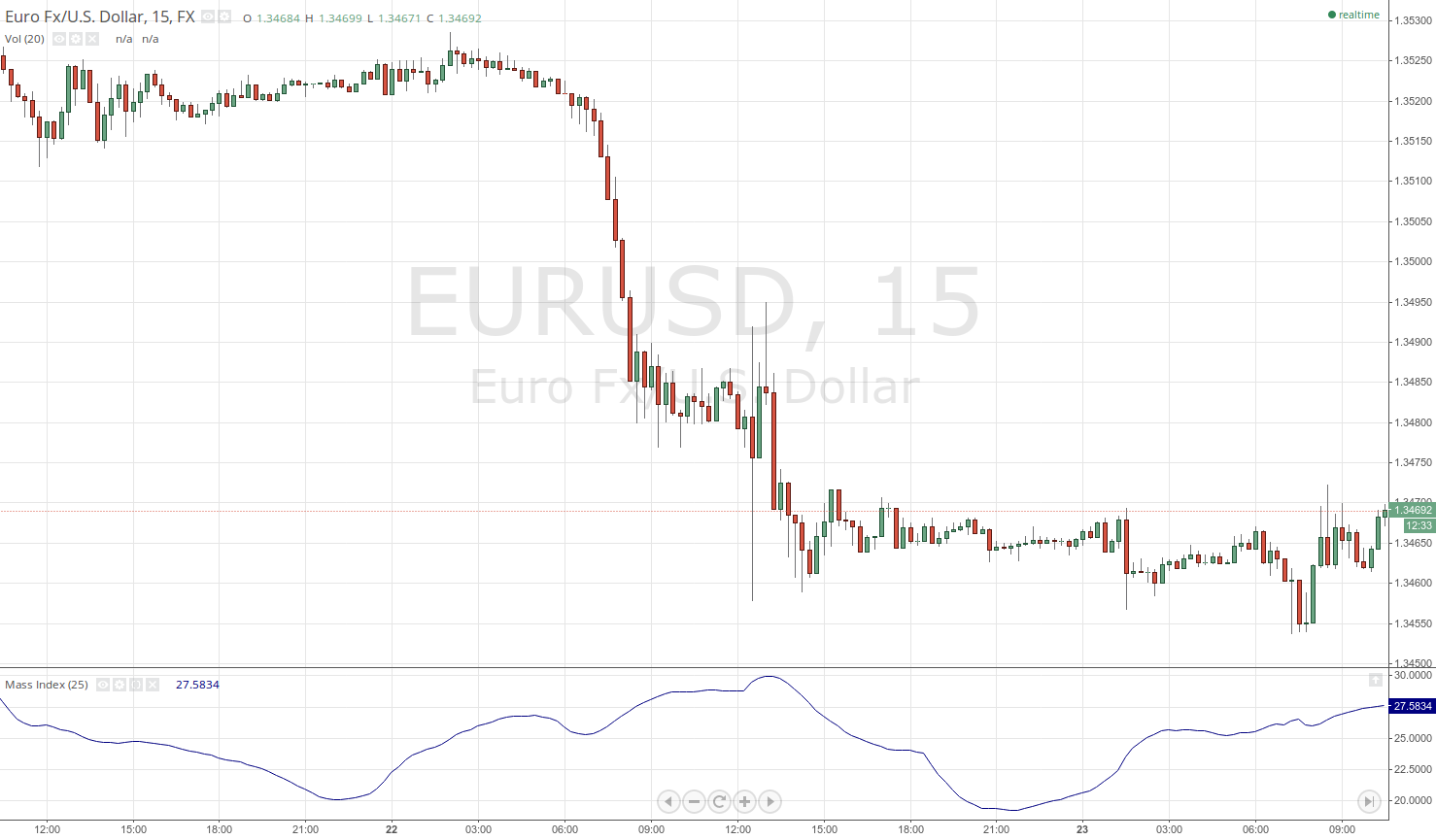# Mass Index

## Mass Index

### This lesson will cover the following

• Definition
• Calculation
• Interpretation

Developed by Donald Dorsey, the Mass Index is a volatility indicator which uses the expansion or the shrinkage of the prices high-low range to identify trend reversals. It rises as the average price range (the difference between the highs and lows) grows, and declines as the price range narrows. However, because the Mass Index takes into account only volatility, it does not have a directional bias, thus it serves only to signal possible trend reversals.The calculation of the Mass Index includes four steps and is based on an EMA, a double EMA (an EMA of the first EMA), a ratio between the two, and a final 25-day moving sum of the ratio. Below you can see the full list of actions needed to estimate the Mass Index:

## Best Forex Brokers for United States

1. Calculate the single Exponential Moving Average as a 9-period EMA of the prices high-low differential.

2. Calculate the double Exponential Moving Average as a 9-period EMA of the 9-period EMA of the high-low differential (basically a 9-period EMA of the EMA in point 1).

3. Calculate the EMA ratio by dividing the Single EMA by the Double EMA (EMA in point 1 divided by the EMA in point 2).

4. Calculate the Mass Index as a 25-period moving sum of the EMA ratio.

Basically, the Single EMA provides the average for the high-low range, which is then smoothed for the second time by the Double EMA. The ratio between the two normalizes the data and shows when the Single EMA grows relative to the Double EMA, while the final 25-period moving summation further irons out the data.

## Logic

The logic behind this indicator is that surging volatility often accompanies direction shifts, thus a spike in the indicators value should be regarded as a possible upcoming trend reversal. However, there must be a certain threshold that would distinguish “normal” volatility from “excessive” volatility, and thus generate an entry signal when the latter is at hand.

The indicator usually ranges around the mid 20s. According to its creator, the Mass Indexs most important key point and entry level is when its value exceeds the level of 27 and afterwards drops back below 26.5 (when you are using a 25-period sum of the EMA ratio). Here is how the indicator and the pattern look like.The logic behind this move, which Dorsey refers to as the “reversal bulge” is that the market has performed a steep enough move in one direction (thus exceeds the level 27, regarded as a buy/sell climax) and then stands a higher chance of reversing or at least retracing. As soon as the reversal effectively occurs and is confirmed by the indicator dropping below 26.5, an entry signal is generated in the direction of the new trend.

However, using the Mass Index solely to decide whether or not to enter against the old trend and in the direction of the allegedly new one is not wise. Once the reversal bulge is complete (Mass Index retreats below 26.5), the trader should use other technical analysis tools to confirm the direction of the new move and whether it is strong enough to actually reverse the old trend. Also, in some cases the indicators length may need tweaking as the level of 27 may be too high for times of low volatility. In these conditions the Mass Index will hardly produce any signals, thus different markets may require adjustments.